# PC/CP320 Physical Computing

## Introduction to Operational Amplifiers

### Objectives

An operational amplifier or op-amp is a high gain DC amplifier; the operational portion of the name derives from its original application for performing mathematical operations in analog computers. The op-amp is an analog device that is very widely used in both analog and digital design because its operating characteristics can be significantly changed by connecting different external components.

The objectives for this lab are:

• to investigate the behaviour of an op-amp in an open-loop configuration - the comparator
• operation of inverting and non-inverting inputs
• impact of op-amp gain
• limitations imposed by supply voltages
• to investigate the behaviour of an op-amp in one of the many closed-loop configurations - the inverting amplifier
• limitations imposed by supply voltages

### Equipment

• textbook
• bench supply, oscilloscope, function generator
• for open-loop circuit and inverting amplifier use the LM741:
data sheet from local server.

### Procedure

#### The comparator - the op-amp in an open-loop configuration

1. Operational amplifiers are differential amplifiers that amplify the difference in voltage between the two input connections or Vo = Av (V+ - V- ) where Av is the voltage gain.

Using the LM741, construct the open-loop comparator circuit shown.
Since you will be having negative supply voltages that are not the same as ground, use white wires for the negative supply voltage. There are white alligator clips to help with this.

Use ±12V bench (i.e. fixed) supplies for the supply voltages (note the above caution about polarity).
(Note that the diagram does not explicitly show the supply voltages for the op amp.)

Don't confuse the positive supply and the non-inverting input, or the negative supply and the inverting input. Always ensure you know which one you are talking about.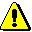Precautions should be taken to insure that the power supply to the operational amplifier never becomes reversed in polarity. The input voltage at the positive supply pin must be greater than the input voltage at the negative supply pin. If polarity is reversed to the IC, the internal conductors can fuse and destroy the chip.

Use the two variable dc supplies for V1 and V2 (from the supply on top of the bench).Never apply inputs before establishing supply voltages.

Don't confuse the positive supply and the non-inverting input, or the negative supply and the inverting input. Always ensure you know which one you are talking about.

Monitor Vout with a digital voltmeter.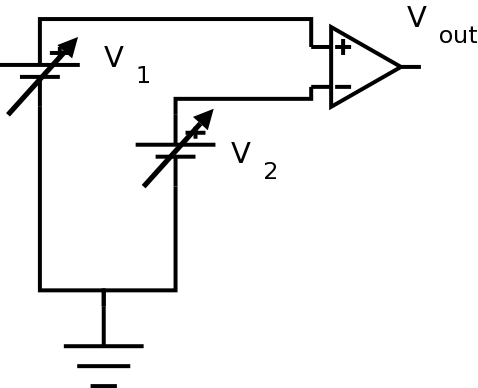Sketch the circuit in your lab notebook. Since you will be doing a lot of circuit variations, it is important to get into the habit of always sketching the circuit in your lab notebook. Always include the supply voltages.

1. Set V1 to 1 volt, and V2 equal to 0 volts. Note Vout.
2. Increase V2 slowly to 2 volts, while watching Vout. Don't change V1.
If you notice anything happen to Vout, record what happens and the value of V2.
3. Now leave V2 at two volts, and increase V1 slowly to 3 volts, while watching Vout.
If you notice anything happen to Vout, record what happens and the value of V1.
4. Repeat the pattern of the last two steps, leapfrogging V1 and V2 until they reach the positive supply voltage or until you see a pattern emerge. What pattern did you observe in Vout?

2. Since Vo = Av (V+ - V_ ), what output would you expect if you had unity voltage gain, i.e. Av = 1? Explain.

Knowing this, what can you say about Av for this op-amp, e.g. is it much less than 1, is it less than 1, equal to 1, greater than 1, much greater than 1? Explain your reasoning.

Demonstrate your results (i.e. explain why this is called a "comparator") to the lab supervisor.

#### The inverting amplifier - one of the many closed-loop configurations

1.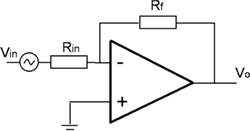An inverting amplifier circuit produces an amplified output signal that is 180° out of phase with the input signal.

Using an LM741, construct the closed-loop inverting amplifier circuit shown to the left. Use ±12V for the supply voltages. Use a feedback resistor, Rf, of 10 kOhms and an input resistor, Rin, of 1 kOhms. When you sketch the circuit in your lab notebook, note the feedback and input resistor values.

In order to show that the circuit works as expected, you'll need to display both the input and output at the same time.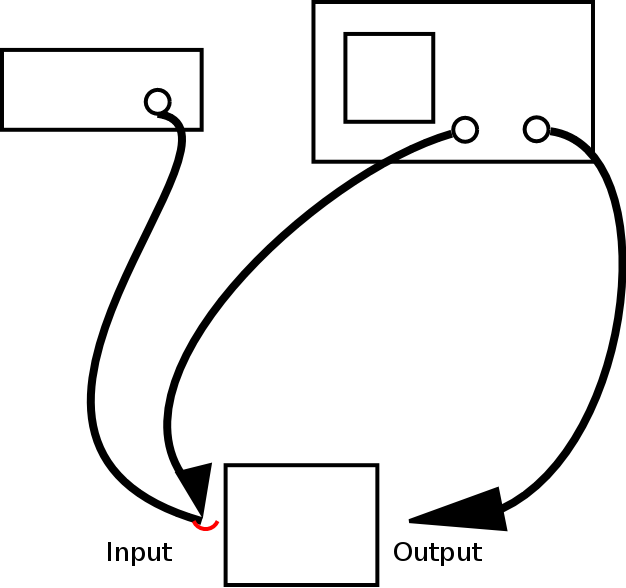Drive the amplifier with a 1 kHz sine wave with a DC offset of zero. Start with a small amplitude and increase the amplitude; note the signal behaviour as the amplitude increases. Sketch input and output; include measurements of amplitude.

At what point does the signal saturate (output signal will no longer look like a sine wave)? For the remainder of this exercise, use a reasonable amplitude (not too small but do not saturate the output).

2. Theoretically, Vo = Av (V+ - V_ ) where Av is the open-loop voltage gain. The closed-loop gain is a function of the feedback resistor and the input resistor. For the circuit in the previous question (nonsaturated state), what closed-loop gain did you observe? How does this relate to the resistors used in the circuit?

3. What maximum output swing did you observe? What is this as a function of the rails?

4. Since you know the equation (question 2) and you know the supply voltages you used, you can calculate the point at which saturation would occur, i.e. for what value of Vin? Show the calculation.

5. Measure the voltage at the inverting input. Sketch the circuit and indicate how you are doing this measure.

The inverting input is said to be at virtual ground. A virtual ground is a voltage ground because the point is at 0V; however, it is not a current ground because it cannot sink any current.

6. Change the supply voltage to +5V/-12V. Sketch your circuit and show both the input and output voltages. Do you understand why the supply voltages are called the rails? Note the relationship of Vo to the supply voltages; does Vo reach the rails?

7. Change the supply voltage to +5V/0V. (What is this configuration called?) Sketch your circuit and show both the input and output voltages. Is this consistent with what happened in the previous item? What does this illustrate about using inverting amplifiers in single supply configurations?

8. Return to a supply voltage of ±12V. Try sine waves of different frequencies; at some point the amplifier will stop working properly. Note what happens and when it happens.

9. What happens if you try different resistor values? Hold either the feedback or input resistor constant and change the other resistor. What is the relationship between the resistors and the gain? Do you see the voltage divider formed by Rin and Rf?

Demonstrate the inverting amplifier circuit to the lab supervisor. Be prepared to explain the circuit's behaviour.
In order to show that the circuit works as expected, you'll need to display both the input and output at the same time.
You do not have to keep the circuit past the demonstration.
Have the lab supervisor sign your lab notebook before you leave the lab.

## Resources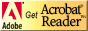If you need to update a browser, you might try Firefox which is
• free
• open source
• available for several platformsSince this page uses cascading style sheets for its layout, it will look best with a browser which supports the specifications as fully as possible.

If you are looking for an office package, with a word processor, spreadsheet, etc., you might try LibreOffice which is
• free
• open source
• available for several platforms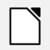Go to the main page for the Department of Physics and Computer Science.

Wilfrid Laurier University